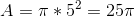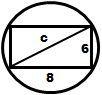High School Math : Circles

Example Questions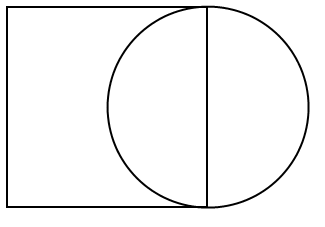As illustrated above, a square has one side that is the diameter of a circle. If the area of the square isunits, what is the area of the circle?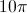units squared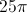units squared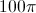units squared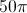units squared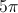units squaredunits squared

Explanation:

Find the length of one side of the square by using the formula for the area of a square,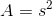, whereis the length of one side, with the given information.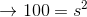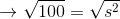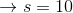units

Since the side of the square forms the diameter of the circle, half of the side will be the length of the circle's radius. The radius is thus calculated as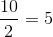units.

Now use this radius in the equation for the area of a circle,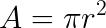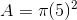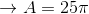units squared

Example Question #41 : How To Find The Area Of A Circle

A 12x16 rectangle is inscribed in a circle. What is the area of the circle?

10π

50π

100π

120π

90π

100π

Explanation:

Explanation: Visualizing the rectangle inside the circle (corners touching the circumference of the circle and the center of the rectangle is the center of the circle) you will see that the rectangle can be divided into 8 congruent right triangles, with the hypotenuse as the radius of the circle. Calculating the radius you divide each side of the rectangle by two for the sides of each right triangle (giving 6 and 8). The hypotenuse (by pythagorean theorem or just knowing right triangle sets) the hypotenuse is give as 10. Area of a circle is given by πr2. 102 is 100, so 100π is the area.

Example Question #71 : Circles

A circle has a radius of 5 inches.  What is the area of the circle in square inches?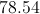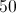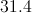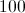Explanation:

The area of a circle is found by squaring the circle's radius and multiplying the result by.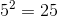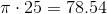Example Question #72 : Plane Geometry

A circle is inscribed in a square whose side is 6 in. What is the difference in area between the square and the circle, rounded to the nearest square inch?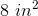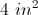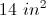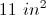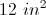Explanation:

The circle is inscribed in a square when it is drawn within the square so as to touch in as many places as possible. This means that the side of the square is the same as the diameter of the circle.

Let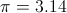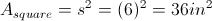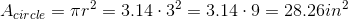So the approximate difference is in area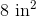Example Question #92 : Geometry

Find the area of the shaded region: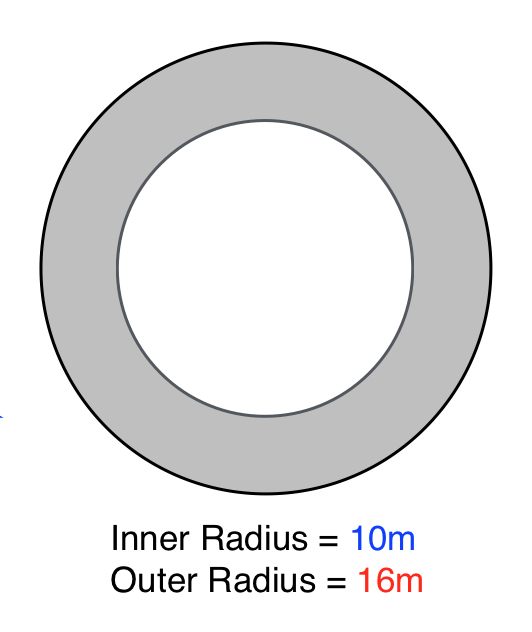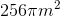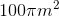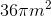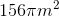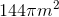Explanation:

The formula for the area of a circle is: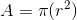whereis the radius of the circle.

In order to find the area of the shaded region, you must subtract the area of the inside circle from the area of the outside circle.

Therefore, the formula becomes: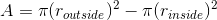Plugging in our values, we get: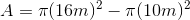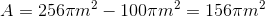Example Question #41 : How To Find The Area Of A Circle

Find the area of a circle with a circumference of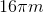.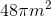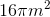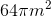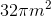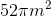Explanation:

The formula for the circumference of a circle is:,

whereis the radius of the circle.

To solve for the radius, plug in our existing values: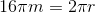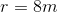The formula for the area of a circle is: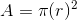,

whereis the radius of the circle.

Plugging in our values, we get: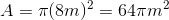Example Question #94 : Geometry

Find the area of the following circle: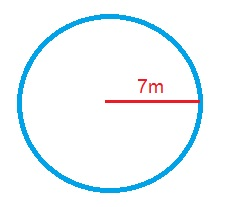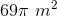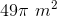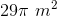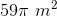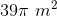Explanation:

The formula for the area of a circle is,

whereis the radius of the circle.

Plugging in our values, we get: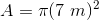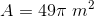Example Question #23 : How To Find The Area Of A Circle

Two equal circles are cut out of a rectangular sheet of paper with the dimensions 10 by 20. The circles were made to have the greatest possible diameter. What is the approximate area of the paper after the two circles have been cut out?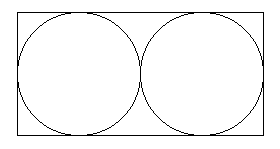16

43

23

56

43

Explanation:

The length of 20 represents the diameters of both circles. Each circle has a diameter of 10 and since radius is half of the diameter, each circle has a radius of 5. The area of a circle is A = πr2 . The area of one circle is 25π. The area of both circles is 50π. The area of the rectangle is (10)(20) = 200. 200 - 50π gives you the area of the paper after the two circles have been cut out. π is about 3.14, so 200 – 50(3.14) = 43.

Example Question #24 : How To Find The Area Of A Circle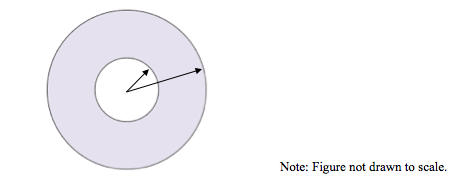Kate has a ring-shaped lawn which has an inner radius of 10 feet and an outer radius 25 feet. What is the area of her lawn?

125π ft2

275π ft2

175π ft2

325π ft2

525π ft2

525π ft2

Explanation:

The area of an annulus is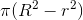whereis the radius of the larger circle, andis the radius of the smaller circle.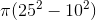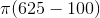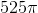Example Question #25 : How To Find The Area Of A Circle

A 6 by 8 rectangle is inscribed in a circle. What is the area of the circle?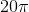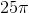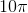The image below shows the rectangle inscribed in the circle. Dividing the rectangle into two triangles allows us to find the diameter of the circle, which is equal to the length of the line we drew. Using a2+b2= c2 we get 6+ 82 = c2. c2 = 100, so c = 10. The area of a circle is. Radius is half of the diameter of the circle (which we know is 10), so r = 5.